# 11-Plus Exam Illustrations - Maths Quiz - Area of Rectilinear and Curved Shapes (Questions)

Area of Rectilinear Shapes

Don't be put off by the term 'rectilinear' - it just means that the angles are 90° (right angles) so the calculations are straightforward. This section includes squares, rectangles and composite shapes made up from more than one put together.

To find the area of a square, multiply the length of one side by itself. For example, a 7cm square has an area of (7 x 7) 49cm2.

Rectangles require you to multiply the length by the width.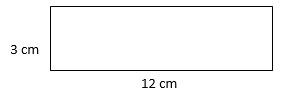The area of this rectangle is (3 x 12) 36cm2.

The area of a composite shape is best worked out by breaking it into component rectangles or squares. As long as you have enough measurements to work out the length and width of each element, you can solve these sorts of questions.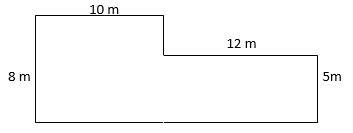This shape can be split into two separate rectangles and the area of both added to give you the answer. In this example, we can make a 10 x 8 rectangle and a 12 x 5 rectangle, thus: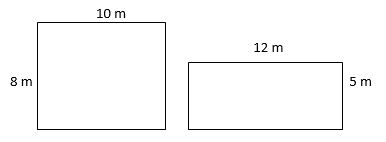The two rectangles have areas of (10 x 8) 80m2 and (12 x 5) 60m2 so their combined area is 140m2.

Area of Curved Shapes

Sometimes your child will be confronted with questions which show a very loosely-drawn shape on a grid and they will be asked to estimate the area or perimeter of it. The key here is not to get hung up on the precision that is required for rectilinear shapes and do as asked - ESTIMATE.

Let's look at such a question: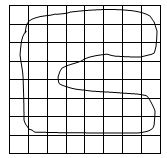What is the area of the shape in the grid above? Estimate how many centimetres squared it covers, given that it is drawn on one-centimetre square paper.

The easiest way to do this is to 'tick off' the whole squares with a physical symbol or by colouring them in. Count these up to give a total of WHOLE squares.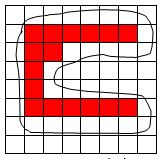There are sixteen whole squares.

Next, pair up squares which appear to add up to provide a whole square. In this case, the ones on the left are very small while the ones on the top are quite large. Added together, they make whole squares.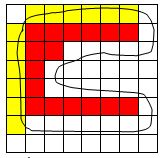Now there are six more squares to add to the total.

If you continue with this process you can pair up all the remaining squares to make whole ones or, if you end up with lots of small ones or lots of large ones, group them together to make one or two whole squares. For example, the squares marked in green may not neatly fit in with others elsewhere to make complete squares. For these, it's easier to look at the amount of space outside of the shape and take it away from the total number of squares being considered.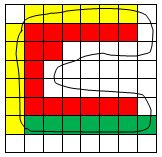Of the seven squares, it seems like two squares' worth of space is not covered by the shape. I would say 'five whole squares' for this area and add it to the running total. Precision is impossible.

With all the pairing up and the running total being carefully maintained, you should end up with an approximate total of 35 square centimetres. There will always be a margin of error in these questions and the likelihood of having options such as 34 or 35 or 36 cm2 is remote. These are not set out to trick people but to get a reasoned response.